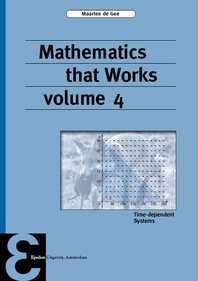# Epsilon uitgaven Mathematics that Works 4Voorzijde37,00
Met 5% studentenkorting
Voor 35,15
Levertijd: 48 uur
ISBN: 9789050411615
Uitgever: Epsilon Uitgaven
Verschijningsvorm: Paperback / softback
Auteur: Maarten de Gee
Druk: 1
Pagina's: 400
Taal: Engels
Verschijningsjaar: 2017
NUR:

From ancient times, mathematics has developed side-by-side with physics; only in the last two hundred years they came apart as different branches of science. Extensive use of mathematics in other sciences, such as biology, chemistry, economics, pharmacology, environmental and social sciences is even more recent. Nowadays, knowledge of mathematical techniques is indispensable also for students and researchers in these fields.

The series of textbooks “Mathematics that Works” presents mathematical methods with a sharp focus on applicability. There is an ample supply of worded-out examples and instructive exercises. The techniques are illustrated in applications from various branches of science; here, the connection between the mathematical method and the application is equally important as the mathematics itself. Thus the way the students digest the matter is aimed at practical usability within their own field, and skills take priority over the mathematical abstraction level. By connecting mathematics to its applications the usage of mathematics in the applied sciences is facilitated.

This volume treats time-dependent processes that can be modelled with ordinary differential equations. This text is intended as a second course; the reader is assumed to be familiar with the basic concepts from calculus and linear algebra. The level of difficulty is somewhat higher than in the previous volumes; the applications are more advanced, and the mathematical modelling has more emphasis. Thus the technical and computational skills shift to the second place. It is assumed that modern engineers and scientists supplement their conceptual abilities on the computational level with computer packages as Matlab™, Maple™ or Mathematica™.

Lees meer